# Goldengorin

## What is the smallest number of disjoint spanning trees made a graph Hamiltonian ★★

Author(s): Goldengorin

We are given a complete simple undirected weighted graph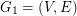and its first arbitrary shortest spanning tree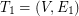. We define the next graphand find onthe second arbitrary shortest spanning tree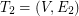. We continue similarly by finding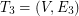on, etc. Let k be the smallest number of disjoint shortest spanning trees as defined above and letbe the graph obtained as union of alldisjoint trees.

Question 1. What is the smallest number of disjoint spanning trees creates a graph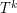containing a Hamiltonian path.

Question 2. What is the smallest number of disjoint spanning trees creates a graphcontaining a shortest Hamiltonian path?

Questions 3 and 4. Replace in questions 1 and 2 a shortest spanning tree by a 1-tree. What is the smallest number of disjoint 1-trees creates a Hamiltonian graph? What is the smallest number of disjoint 1-trees creates a graph containing a shortest Hamiltonian cycle?

Keywords: 1-trees; cycle; Hamitonian path; spanning trees